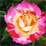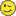PSoC™ 4Level 4Level 4

# Using UDB datapath Parallel Input (PI) as an ALU constant

I wanted to do this, but could not find any reference (one way or the other) to being able to use the Parallel Input (PI) to the datapath as a constant for calculations with the ALU (e.g. add or subtract), so I decided to give it a try because this would be very helpful in one of the things I'm trying to do with UDBs.

The answer? Yes, you can use the PI as a constant! I have attached a simple project to demonstrate this (I did it on a CY8CKIT-049-42xx, you'll need to reconfigure the Bootloadable component with your elf/hex files for it if you try to compile it for the same target).

Since there's not a lot of documentation on using the PI, much less in this manner (I really couldn't find anything that mentioned using PI as a constant, but my search skills may not be 100%), I thought I should document what I did and share it with others. Following the general steps outlined in AN82156 "Designing PSoC Creator Components with UDB Datapaths", section A.5 "Project #5 – Parallel In and Parallel Out", I created a component symbol, added a parameter called "PI_Value" that I set to 5 as default, and created a blank Verilog file for it. The parameter constant was added automatically when the Verilog file was created from the symbol editor, fyi. I then used the Datapath Configuration Tool per the instructions in AN82156 and created a blank cy_psoc3_dp datapath instance in the Verilog file. In the tool, first enabled the PI_DYN bit, then set it to EN. I created two datapath instructions: one to add the value of PI to the value of A1 and then store it back in A1 (FUNC = ADD, SRCA = A1, SRCB = A1, A1_WR_SRC = ALU, and CFB_EN = ENBL... the last of which dynamically selects PI as the input for SRCA to the ALU rather than A0 or A1 when the static PI_DYN bit is EN), and the other to just pass the value of A1 through the ALU (with FUNC = PASS, SRCA = A1, SRCB = A1, CFB_EN = DSBL). My Verilog then just flips between the two states every clock. The idea was that the first state would add 5 to A1 every time it ran, and that's what it does (the second state does nothing, but I wanted to have something heading into different states to make it a bit more representative). To finish up the datapath configuration in the Verilog file, I made the following assignments: .clk(Clock), .cs_addr( { 1'b0, 1'b0, next_state } ), .pi(PI_Value), and .po(po), where next_state was a simple flip-flop "reg next_state;"). So .pi() was passed the constant I had defined at the component level. There's a bit of wiring around the Parallel Output (PO) because I have a little LED board I made so I could watch the state transitions on port P2[7:0] (and that's why the frequency is so slow as well... so I could easily count the binary to make sure it was increment by 5). Note, writing ".po({Out7,Out6,Out5,Out4,Out3,Out2,Out1,Out0});" also seemed to synthesize fine and saved that extra wiring and assign statements (I didn't test it out on hardware though). I was trying to emulate the style of AN82156.

So, there were two things I wanted to mention before wrapping up. The first is that the way PO works is not immediately clear from the basic datapath diagram that appears in a lot of the documentation (e.g. the TRM, Rev *H, Figure 16-6 "Datapath Top-Level"). The implication is that PI, A0, and A1 are multiplexed onto SRCA to the ALU, and that PO is connected to the SRCA connection itself (this is literally how it's drawn in that figure). That was one of the reasons why I initially made two different datapath states: because I thought that in the ADD state, since I was routing PI to SRCA, that I would see it on PO. I added the second state so A1 would be applied to SRCA and PO would then alternate between PI and A1 (I needed to see A1, but seeing the constant every second cycle was fine). It didn't work that way... all I got out of PO was the value for A1. I had to dig deep into the TRM to find the answer, but in section 16.2.2.8 of the TRM (Rev. *H) "Datapath Parallel Inputs and Outputs", there is another diagram (Figure 16-25 "Datapath Parallel In/Out") that shows the insides of the SRCA multiplexer. In this diagram, it is shown that there are two multiplexers: one that selects between A0 and A1 that feeds into a second multiplexer whose other input is PI. PO is connected to the output of the first multiplexer... so that PO can never see the value of PI, and even when PI is being used, PO will be connecting to either A0 or A1 only. Mystery solved. But ugh.

Lastly, there seems to be a couple of bugs in AN82156 "Designing PSoC Creator Components with UDB Datapaths", section A.5 "Project #5 – Parallel In and Parallel Out". It assigns two bits to a one bit register ("state"), and then suggest a non-blocking assigning of the PO value out of the datapath to the component's output:

`// From AN82156 ... bugs?reg state;wire[7:0] po;localparam STATE_LOAD = 2'b00;localparam STATE_ADD = 2'b01;always @( posedge clk )begin  case (state)    STATE_LOAD:      begin        state <= STATE_ADD;        /* we must latch the PO value here, because in the next state PO is not valid*/        Parallel_Out <= po;    end  STATE_ADD:    begin      state <= STATE_LOAD;    end  endcaseend`

But Warp would complain (rightfully so I think) when I tried it with my component, that Out7 through Out0 were "not a register type", and could not be assigned in that way. I may be doing something wrong (let me know if you know what it is), since I am no expert at this.

`// Does not work!!!wire [7:0] po;reg next_state;always @ (posedge Clock)begin  case(next_state)    1'b0:      begin        Out7 <= po;        Out6 <= po;        Out5 <= po;        Out4 <= po;        Out3 <= po;        Out2 <= po;        Out1 <= po;        Out0 <= po;        next_state <= 1'b1;      end    1'b1:      begin        next_state <= 1'b0;      end  endcaseend`

In my case at least, fIguring out how to use UDBs is hard enough without these couple of additional issues.One last thought (I haven't tried this yet, it's next on my list). Since the the SUB function of the ALU is "SRCA - SRCB", if A1 is SRCB, it is not possible to subtract PI from it (and store it back in A1, for instance). However, if PI is stored as an 8-bit 2's complement negative number and is added to the A1 register instead of subtracted, then the result will be that the PI value is effectively subtracted from the A1 value. I need to figure out the flags, but this seems like it should work fine. Again, I haven't tried it, but maybe setting the parameter as an int8 instead of a uint8 will allow negative numbers and will encode it properly.

Edit 2020/09/10: I did try what I suggested with the 2's complement arithmetic and it worked like a charm. For instance, to subtract 5 by using the ADD function of the ALU, I set PI to  -5 in 2's complement (".pi(8'b11111011)") in the datapath setup, and tried ADDing it with a few values like 10, 5, and 3, and I got the correct answers (which was expected). I wanted to know what the flags were set to so I could figure out the best way to do 16-bit arithmetic on a single 8-bit datapath, so I used A1 as the MSB and A0 as the LSB. What is extra nice, is the state of the carry out bit is 0 if the "addition" of the 2's complement PI to A0 results in a negative number, and 1 if not. I used the registered carry flag input to a DEC command on the MSB register (A1), and it performed the operation A1 <- A1 - 1 + carry (carry is 1 if ADD PI + A0 is a positive number, so the DEC A1 command with the registered carry option gives A1 <- A1 - 1 + 1, so A1 doesn't get decremented; but if PI > A0 and the operation ends up with a negative result, the operation is A1 <- A1 - 1 + 0, which decrements A1). So this can be used for 16-bit arithmetic on the 8-bit ALU with two cycles! I double-checked the SUB command on the ALU, subtracting A0 <- A0 - A1 for instance, and the carry flag (which the spec says is "inverted" for SUB results, but I wasn't sure what that meant exactly), let's you use the DEC A1 command with registered carry again to do proper 16-bit math. Just fyi.

Edit 2020/09/14: Short summary: this technique uses a macrocell! Long summary: I was working on my component that used the above technique and I kept seeing an extra macrocell being used. I had declared 4 registers, but 5 macrocells were being allocated to the design and it was driving me nuts trying to figure out what was causing it. I had assumed I had messed up an if or case statement or something and was causing an implicit latch instantiation, but nothing I did seemed to make any difference (and from what I can tell, Warp doesn't care if you're missing a final else statement, or if you haven't specified all possible cases in a case statement... it doesn't create implicit latches like other synthesizers in those cases it seems). I eventually broke down and started rummaging in the PSoC Creator file output and got my answer. It turns out the extra macrocell was used to provide the value to the Parallel Input (which is why I mention it here). In the "codegentemp" there was a file <component_name>_p.vh2 that is the VHDL translation of the Verilog code it looks like. In it, there was a macrocell definition called "__ONE__". The only thing this macrocell does is supply logic 1s to the appropriate bits in the value provided to the Parallel Input. If I set .pi(0) in the datapath instantiation, the macrocell goes away. I had thought that the constant bits would be supplied through a connection with the routing channels (like there was a magic source of 1s and 0s available on it), but it seems that a macrocell in the UDB is needed to supply that logic level. Fair enough, but it's good to know that one of the macrocells will be used if you use the Parallel Input to supply a constant value. I have enough to spare, but was worried it was something more potentially vexing. I don't know where the 0s are coming from (the PI bits that were logic 0 were not connected in the netlist to anything), but I'm not too concerned, it works fine. In the code below, it was set as ".pi(8'b11111011)".

`   \TEST_COMP:DP_INST\:datapathcell        GENERIC MAP(            [ ... bunch of config deleted ...]            uses_p_out => '0',            clk_inv => '0',            clken_mode => 1)        PORT MAP(            clock => Net_35_digital,            cs_addr_2 => \TEST_COMP:state_2\,            cs_addr_1 => \TEST_COMP:state_1\,            cs_addr_0 => \TEST_COMP:state_0\,            ce0_comb => \TEST_COMP:lsb_equal\,            z0_comb => \TEST_COMP:lsb_zero\,            ce1_comb => \TEST_COMP:msb_equal\,            z1_comb => \TEST_COMP:msb_zero\,            p_in_7 => __ONE__,            p_in_6 => __ONE__,            p_in_5 => __ONE__,            p_in_4 => __ONE__,            p_in_3 => __ONE__,            p_in_1 => __ONE__,            p_in_0 => __ONE__,            busclk => ClockBlock_HFClk);    __ONE__:macrocell        GENERIC MAP(            eqn_main => "1'b0",            regmode => 0,            clken_mode => 1)        PORT MAP(            q => __ONE__);`

I am getting closer to being done now that this is resolved as well.ModeratorModerator

# Re: Using UDB datapath Parallel Input (PI) as an ALU constant

Thank you for your contribution to the community and the example project.

Best Regards

Ekta